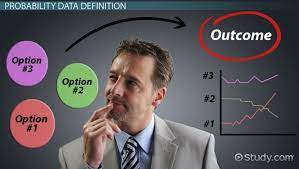Probability is a branch of applied mathematics that deals with the analysis of random events. The outcome of a random event cannot be predicted before it happens; it might be one of several possibilities. This eventual outcome is thought to have been determined by chance. This concept of uncertainty is why the subject of probability is quite important and has different applications in our daily lives and business.

There are numerous instances in real life where we may need to make predictions about how something will turn out. The outcome of an event may or may not be known to us. In general, probability has many wonderful uses in games, in business to make forecasts based on likelihood, and in this emerging branch of artificial intelligence.

By simply dividing the favourable number of possibilities by the entire number of potential outcomes, the probability of an occurrence may be determined using the probability formula.

The number of positive results for an experiment with ‘n’ outcomes can be represented by the symbol x. The probability of an occurrence may be calculated using the following formula.

Probability (Event) = Favourable Outcomes/Total Outcomes = x/n

Some probability terminologies listed to aid in a better comprehension of probability concepts:

Experiment: A trial or procedure carried out to generate a result.

Sample Space: A sample space is the collection of all potential outcomes of an experiment. Tossing a coin, for instance, has two possible outcomes: head or tail.

Favorable Outcome: An event that has produced an expected outcome. If we roll two dice and receive the total of the two numbers as 4, the possible/favorable possibilities are (1,3), (2,2), and (3,1).

Trial: A trial implies doing a random experiment.

Random Experiment: A random experiment is one that has a well-defined set of results. Tossing a coin, for instance, gives us the option of getting either the head or the tail, but we never know for sure which one will come up.

Event: An event is the whole assortment of results from a random experiment.

Probability Formula

P(A) = Number of favorable outcomes to A/Total number of possible outcomes

P(B) is the probability of an event ‘B’.

n(B) is the number of favorable outcomes of an event ‘B’.

n(S) is the total number of events occurring in a sample space

Addition rule: Whenever an event is the union of two other events, say A and B, then
P(A or B) = P(A) + P(B) – P(A∩B)
P(A ∪ B) = P(A) + P(B) – P(A∩B)

Complementary rule: Whenever an event is the complement of another event, specifically, if A is an event, then P(not A) = 1 – P(A) or P(A’) = 1 – P(A).
P(A) + P(A′) = 1.

Probability formula with the conditional rule: When event A is already known to have occurred and the probability of event B is desired, then P(B, given A) = P(A and B), P(A, given B). It can be vice versa in the case of event B.
P(B∣A) = P(A∩B)/P(A)

Probability formula with multiplication rule: Whenever an event is the intersection of two other events, that is, events A and B need to occur simultaneously. Then P(A and B) = P(A)⋅P(B).
P(A∩B) = P(A)⋅P(B∣A)

#MEMBA11## Next Generation Fixed Wireless Access (NGFWA) for Service Providers: A Viable Alternative to Traditional Fiber-Optic Networks

in## Transforming Nigeria’s Local Government System for Rural Economic Growth and Increased GDP

in